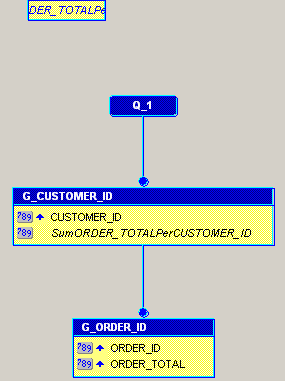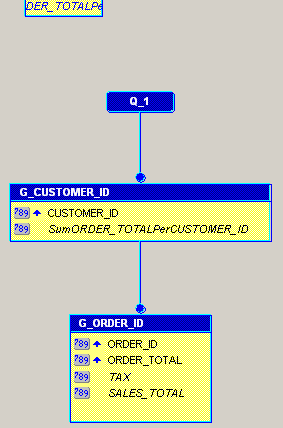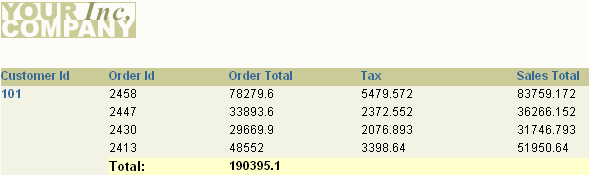# 14.3 Create Two Formula Columns

Frequently, you want to base calculations on values in your data source. One way you can do this is by using formula columns. The steps in this section will show you how to create two formula columns that calculate the following values:

• the tax on each order

• the grand total for each customer, including tax

## 14.3.1 Create a formula column to calculate the tax

To create a formula column that calculates the tax:

1. In Reports Builder, click the Data Model button in the toolbar to display the Data Model view of your report.

The data model should look something like this:

Figure 14-3 Data Model of the Formula ReportDescription of "Figure 14-3 Data Model of the Formula Report"

2. Click the Formula Column tool in the tool palette, then click in the G_ORDER_ID group under ORDER_TOTAL to create a formula column.

Tip:

To view the names of the tools in the tool palette and the toolbar, drag and hold your mouse over each icon and hint text will display describing the icon.
3. Double-click the new formula column object (CF_1) to display the Property Inspector, and set the following properties:

• Under General Information, set the Name property to Tax.

• Under Placeholder/Formula, click the PL/SQL Formula property field to display the PL/SQL Editor.

4. In the PL/SQL Editor, use the template to enter the following PL/SQL code:

```function TAXFormula return Number is
tax number;
begin
tax := :ORDER_TOTAL * .07;
return (tax);
end;
```
5. Click Compile.

6. If no errors display, click Close. If errors display, verify that your code looks exactly like the above code, paying close attention to the column names.

7. Close the Property Inspector.

You have created a formula column that calculates the tax (7%) of each order.

## 14.3.2 Create a formula column that calculates customer order totals

To create a formula column that calculates customer order totals:

1. Repeat Steps 2 and 3 from Section 14.3.1, "Create a formula column to calculate the tax": click the Formula Column tool in the tool palette, then click in the G_ORDER_ID group under TAX. Then, double-click CF_1 to display the Property Inspector.

2. Change the name of the column to `SALES_TOTAL`.

3. Open the PL/SQL Editor and modify your formula so that it looks like this:

```function SALES_TOTALFormula return Number is
sales_total number;
begin
sales_total := :ORDER_TOTAL + :TAX;
return (sales_total);
end;
```
4. Click Compile.

5. If no errors display, click Close. If errors display, verify that your code looks exactly like the above code, paying close attention to the column names.

6. Close the Property Inspector.

You have created a formula column that calculates the total orders of each customer. Your data model should now look something like this:

Figure 14-4 Data Model with Formula ColumnsDescription of "Figure 14-4 Data Model with Formula Columns"

## 14.3.3 Add the formula columns to the report layout

Now that you have created your formula columns, you must add them to your report layout. The easiest way to do this is to return to the Report Wizard.